### Countdown Timer

#### Introduction

In this project, we will make a countdown timer and display it on Magicbit display.

#### Intended Learning Outcome

• You will learn how to make use of functions and use delay node.

First, you need the below nodes.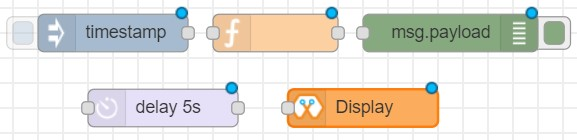Make the following arrangement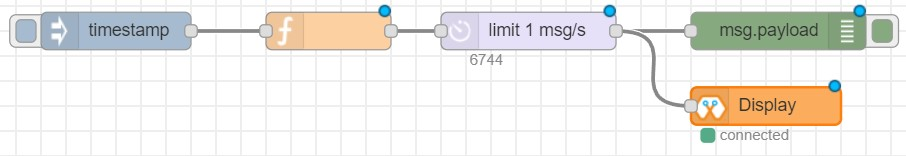#### Inject Node

Double click on the inject node to access its settings. Make the below settings.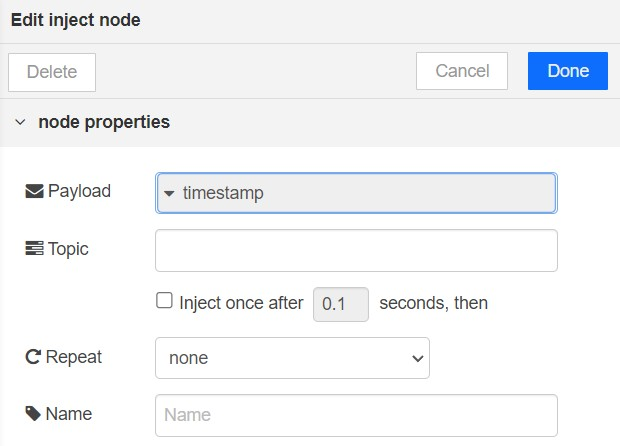#### Function Node

Enter the below code in the Function.

```var H = 1;
var M = 1;
var S = 10;

var seconds = H*3600 + M*60 + S;
for (var i= seconds; i>=0 ; i--) {
if (S===0) {
S = 59;
M--;
}
if (M==0 && H!=0) {
M = 59;
H--;
}
S--;
timer = H+":"+M+":"+S;
}
```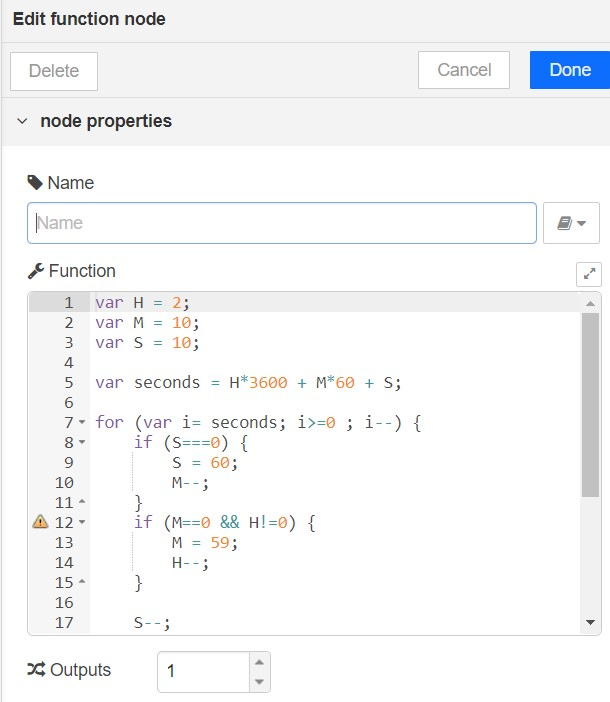You can set the number of hours, minutes, and seconds that you need to countdown under H, M, and S in this code

(See the below example).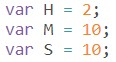#### Delay Node

Make the below settings.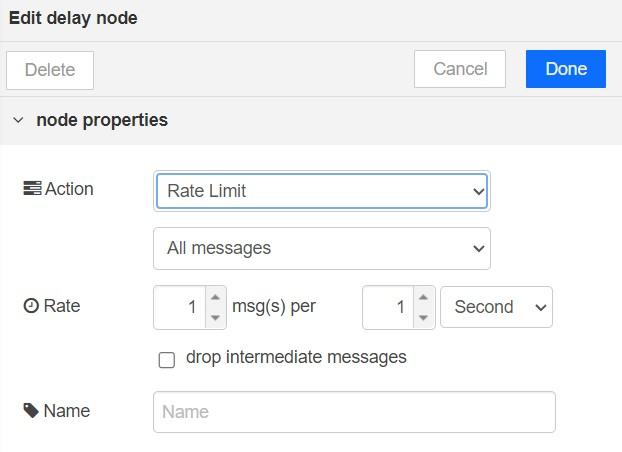#### Display Node

Select the board and set the font size to 2.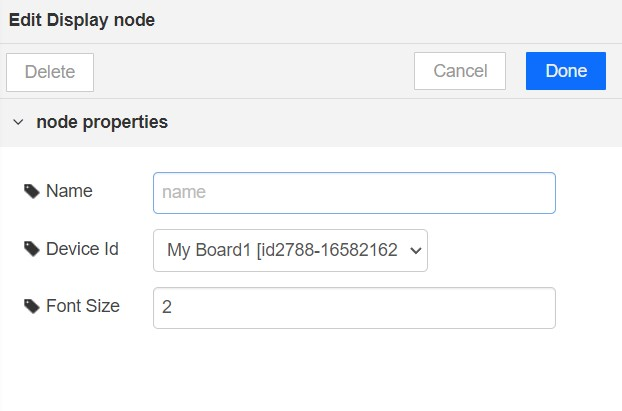Deploy the playground and press on inject node to start the timer.

You will see the Countdown Timer on the Magicbit Display.